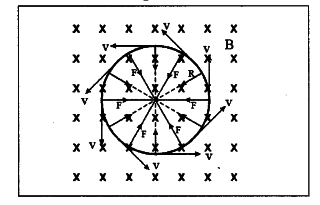# A charged particle q' is moving with a speed V

A charged particle q’ is moving with a speed V perpendicular to the magnetic field of induction B. Find the radius of the path and the time period of the particle.

Let us assume that the field is directed into the page as shown in figure. Then the force experienced by the particle is F = qvB. We know that this force is always directed perpendicular to velocity. Hence the particle moves along a circular path and the magnetic force on a charged particle acts like a centripetal force.
Let r be the radius of the circular path.
We know that centripetal force = m${{v}^{2}} / r q v B = m{{v}^{2}} / r Solving this equation, we get; r = mv / Bq Time period of the particle; T = 2\pi$r / v
Substituting r in above equation, we get
T = 2$\pi$m / Bq